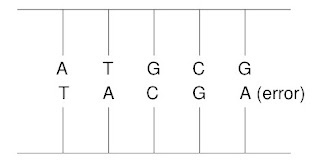## Friday, July 22, 2016

### Error Rates During DNA Copying

Chapter 3 of Intermediate Physics for Medicine and Biology discusses the Boltzmann factor. In the homework exercises at the end of the chapter, we include a problem in which you apply the Boltzmann factor to estimate the error rate during the copying of DNA.
Problem 30. The DNA molecule consists of two intertwined linear chains. Sticking out from each monomer (link in the chain) is one of four bases: adenine (A), guanine (G), thymine (T), or cytosine (C). In the double helix, each base from one strand bonds to a base in the other strand. The correct matches, A-T and G-C, are more tightly bound than are the improper matches. The chain looks something like this, where the last bond shown is an “error.”A DNA molecule containing an error.
The probability of an error at 300 K is about 10−9 per base pair. Assume that this probability is determined by a Boltzmann factor e−U/kBT, where U is the additional energy required for a mismatch.
(a) Estimate this excess energy.
(b) If such mismatches are the sole cause of mutations in an organism, what would the mutation rate be if the temperature were raised 20° C?
This is a nice simple homework problem that provides practice with the Boltzmann factor and insight into the thermodynamics of base pair copying. Unfortunately, reality is more complicated.Biophysics: Searching for Principles, by William Bialek.
William Bialek addresses the problem of DNA copying in his book Biophysics: Searching for Principles (Princeton University Press, 2012). He notes that the A typically binds to T. If A were to bind with G, the resulting base pair would be the wrong size and grossly disrupt the DNA double helix (A and G are both large double-ring molecules). However, if A were to bind incorrectly with C, the result would fit okay (C and T are about the same size) at the cost of eliminating one or two hydrogen bonds, which have a total energy of about 10 kBT. Bialek writes
An energy difference of ΔF ~ 10 kBT means that the probability of an incorrect base pairing should be, according to the Boltzmann distribution, e-ΔF/kBT ~ 10−4. A typical protein is 300 amino acids long, which means that it is encoded by about 1000 bases; if the error probability is 10-4, then replication of DNA would introduce roughly one mutation in every tenth protein. For humans, with a billion base pairs in the genome, every child would be born with hundreds of thousands of bases different from his or her parents. If these predicted error rates seem large, they are—real error rates in DNA replication vary across organisms [see the vignette “what is the error rate in transcription and translation” in Cell Biology by the Numbers], but are in the range of 10−8–10−12, so the entire genome can be copied without almost any mistakes.
So, how is the does the error rate become so small? There are enzymes called DNA polymerases that proofread the copied DNA and correct most errors. Because of these enzymes, the overall error rate is far smaller than the 10−4 rate you would estimate from the Boltzmann factor alone.

Our homework problem is therefore a little misleading, but it has redeeming virtues. First, the error we show in the figure is G-A, which would more severely disrupt the DNA's double helix structure. That specific error may well have a higher energy and therefore a lower error rate from the Boltzmann factor alone. Second, the problem illustrates how sensitive the Boltzmann factor is to small changes in energy. If ΔE = 10 kBT, the Boltzmann factor is e−10 = 0.5 × 10−4. If ΔE = 20 kBT, the Boltzmann factor is e−20 = 2 × 10−9. A factor of two increase in energy translates into more than a factor of 10,000 reduction in error rate. Wow!